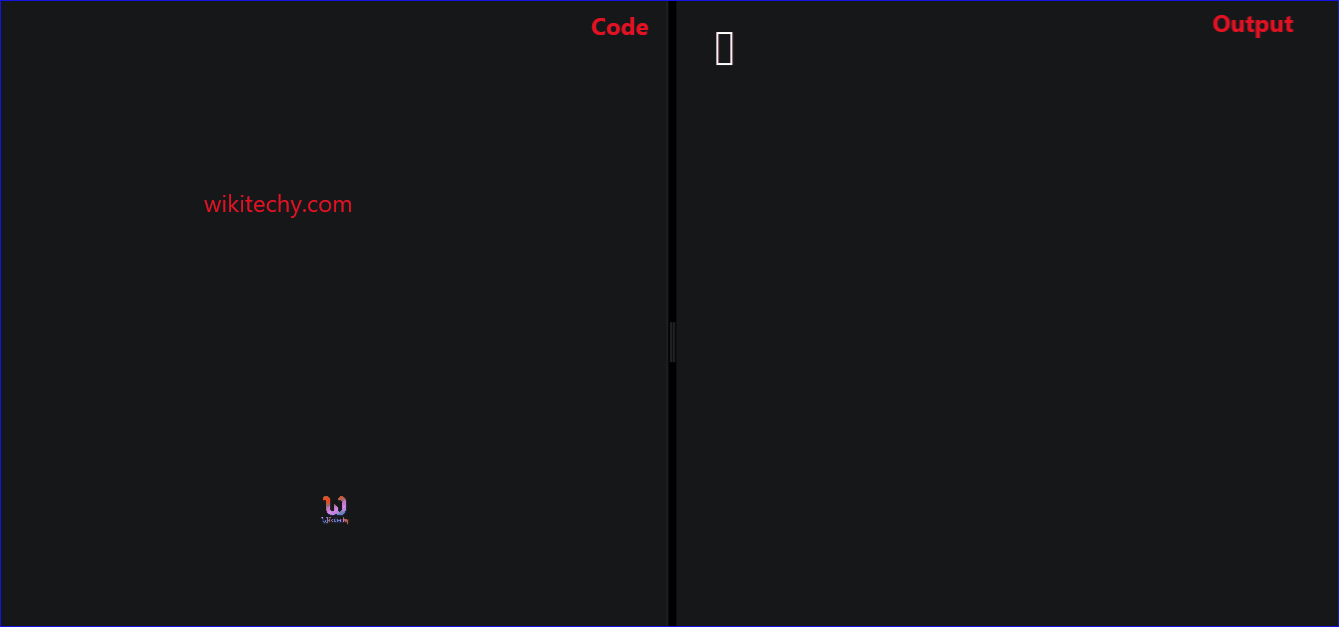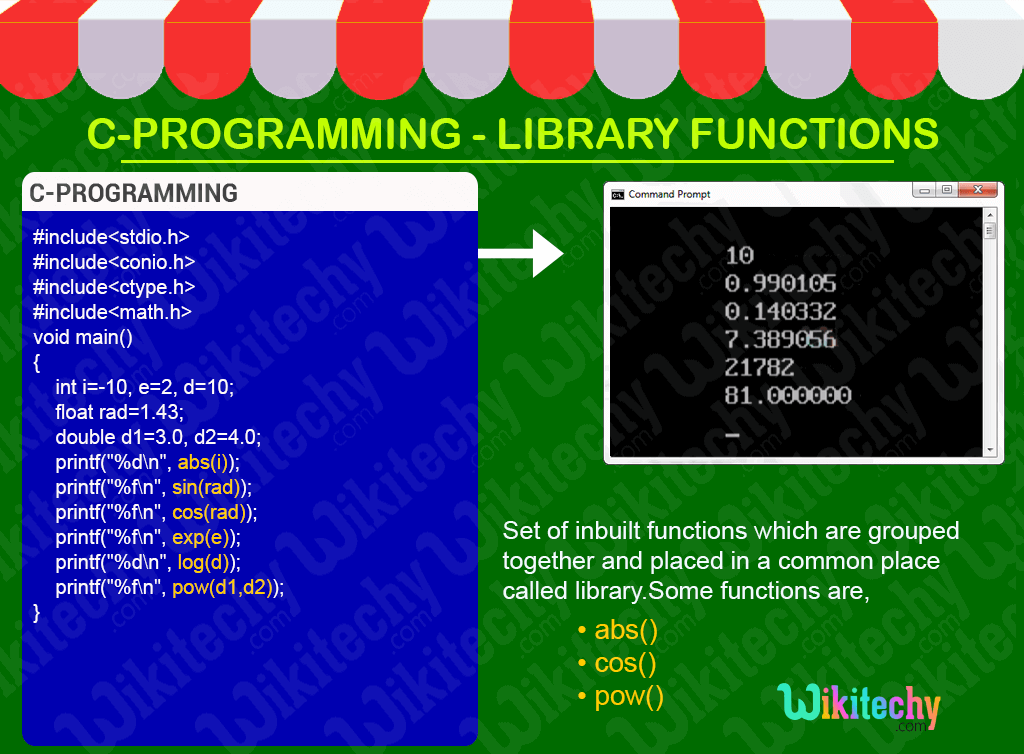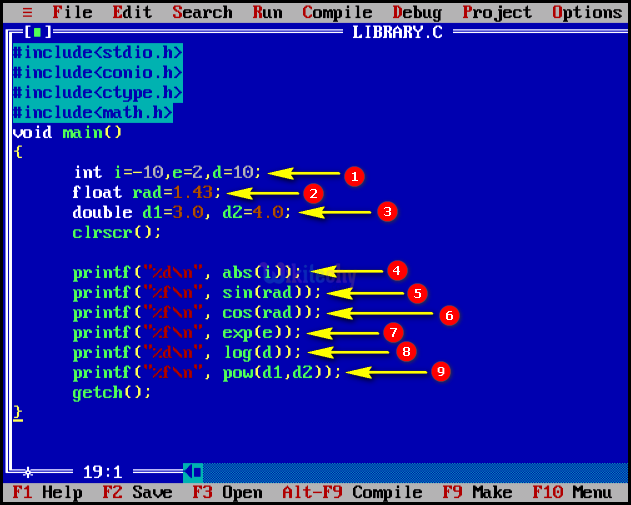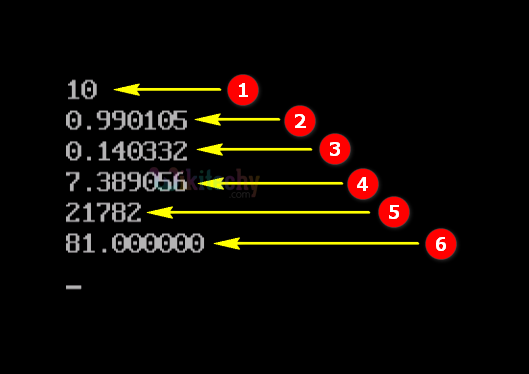# Library Functions in CLearn C - C tutorial - Library functions in c - C examples - C programs

## Library Functions in C - Definition and Usage

• Library functions are inbuilt functions which are grouped together and placed in a common place called library.
• In C- Programming the library functions are accompanied by the number of standard library functions which carry out various useful tasks.
• In general, all the input and output operations and all math operations are implemented by library functions.
• All library functions are declared in many header files which are saved as file_name.h.

## Some of the Library Functions Under Different Header File

• <stdio.h> - Standard Input/Output Function
• <conio.h> - Console Input/Output Function
• <stdlib.h> - Standard Utility Function
• <math.h> - Mathematical Function
• <ctype.h> - Character Type Function
• <assert.h> - Assertion Function
• <string.h> - String Functions
• <time.h> - Date Time Function
• <signal.h> - Signal handling Functions
• <stdarg.h> - Variable Arguments handling Functions
• <error.h> - Error handling Functions
• <locale.h> - Localization Functions
• <setjmp.h> - Jump Functions

C Programming Functions## Sample - C Code

Write a program without using library function

## C Code - Explanation :

1. Here in this statement we declare the variable with its values “i=-10”,” e=2”and “d=10” as integer datatype.
2. In this statement we declare the variable and initialize the value of “rad=1.43”as float datatype.
3. In this statement we declare the variable d1, d2 and initialize the value of d1=3.0 and d2=4.0 as double datatype.
4. Here in this statement we print the absolute value of the variable “i”.
5.6. Here in this statement we print the sin value of the variable “rad”.
7. Here in this statement we print the cos value of the variable “rad”.
8. Here in this statement we print the Exponential value of the variable “e”.
9. Here in this statement we print the log value of the variable “d”.
10. Here in this statement we print the power value of the variable d1 & d2.

## Sample Output - Programming Examples

1. Here in this output we print the absolute value 10 of the variable “i” (where i=-10).
2. Here in this output we print the sin value 0.990105 of the variable “rad” (where rad=1.43).
3. Here in this output we print the cos value 0.140332 of the variable “rad” (where rad=1.43).
4.5. Here in this output we print the Exponential value 7.389056 of the variable “e” (where e=2).
6. Here in this output we print the log value 21782 of the variable “d” (where d=10).
7. Here in this output we print the power value 81.00000 of the variable d1 & d2 (where d1=3.0 and d2=4.0).